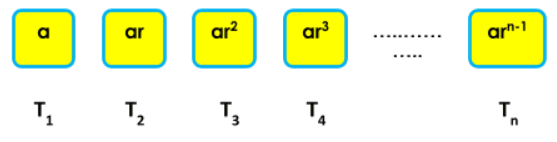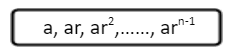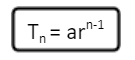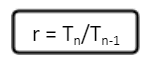Maths
Study Material

# Geometric Progression

A geometric progression (G.P.) is a special type of progression or sequence where in, every term with respect to its succeeding or preceding term bears a constant ratio

6 minutes long
Posted by Kunduz Tutor, 26/10/2021Hesap Oluştur

Got stuck on homework? Get your step-by-step solutions from real tutors in minutes! 24/7. Unlimited.

It can be stated that if the ratio of the successive terms in a sequence is always constant, then that sequence or progression is known as geometric progression, and the constant ratio is called as the common ratio. Moreover, we shall be learning more about the G.P, its formulas and properties, and the various types of geometric progressions.

Author – Ojasvi Chaplot

## Introduction

A geometric progression (G.P.) is a special type of progression or sequence where in, every term with respect to its succeeding or preceding term bears a constant ratio. In order to obtain and find out the next term in the geometric progression, every time we have to multiply with a fixed term referred to as the common ratio. And, if one wants to get the preceding term in the g.p, then we have to just divide the term with the same common ratio as above. Geometric Progression is also referred to as the G.P.

### Common Ratio of Geometric Progression

The common ratio of a G.P, which is the constant ratio, can have a positive or negative value, integer or fractional value.

For Example – A G.P is 2, 4, 8, 16, 32,… therefore, it has a common ratio of 2.

## General Form and General Term of Geometric ProgressionGenerally, the G.P is represented in this form as:This is the sequence of n terms in G.P, where the General Term of the Geometric Progression can be written as:Also, Common Ratio is equal to r, i.e.where, Tn is the general term and Tn-1 is the preceding term.

Here, a is the first term and r is the common ratio of G.P.

## Types of Geometric Progression(s)

The types of G.P are finite and infinite geometric progression(s).

### Finite Geometric Progression (Finite G.P)

The G.P or series which consists of a finite number of terms is known as Finite Geometric Progression. It is a G.P or sequence in which the last term is defined.

For Example – 1, 1/2, 1/4, 1/8, 1/16,…,1/1024 is a finite G.P where the last term is 1/1024.

#### Sum of Finite Geometric Progression

If there are n number of terms in a geometric sequence, i.e. they are finite, then the sum of the finite G.P is calculated by the formula:

Sn = a(1−rn)/(1−r) = a(rn−1)/(r−1) ; for r ≠ 1

And,

Sn = na ; for r = 1

Where, a is the first term, r is the common ratio and n terms in the G.P.

### Infinite Geometric Progression (Infinite G.P)

The G.P or series which consists of an infinite number of terms is known as Infinite Geometric Progression. It is a G.P or sequence in which the last term is not defined.

For Example – 1, 1/2, 1/4, 1/8, 1/16,… is an infinite G.P where the last term is not defined, i.e. it is infinite.

#### Sum of Infinite Geometric Progression

If there are infinite number of terms in a geometric sequence, then the sum of the infinite G.P is calculated by the formula:

S = a/(1−r) ; |r| < 1

Where, a is the first term, r is the common ratio and terms in the G.P.

## Properties of Geometric Progression

The properties of the geometric progression can be classified and listed as follows:

• The first term and the common ratio of a G.P can be any real number.
• The common ratio throughout a G.P remains the same.
• A G.P does not have common difference.
• In a G.P, any term can be expressed as geometric mean (G.M.) of its equidistant terms, i.e. Tr = √( Tr-k x Tr+k).
• Let’s consider a G.P., a, ar, ar2,……, arn-2, arn-1; with a1, a2, a3,….., an-3, an-2, an-1 as terms. Then, a1.an-1 = a2.an-2 = a3.an-3 = ….. and so on.
• If the terms of a G.P are multiplied by a non-zero number K, then the resulting sequence will be in G.P with the same common ratio.
• If the terms of a G.P have a power of a number K, i.e. aK, aKrK, aKr2K,….. then the resulting sequence will be in geometric progression with the common ratio rK.
• The logarithm of each G.P term will result in arithmetic progression with the common difference equal to log r.

## Illustrative Examples

Example-1: Find the 5th term of a geometric progression whose 1st term is 7 and the common ratio is 2.

Solution:

Given that, T1 = a = 7 ———-(1)

And, common ratio, r = 2

Since, the general term of a G.P is Tn = arn-1

Then, T5 = ar4

? T5 = (7).(2)4

? T5 = 7 x 16

? T5 = 112.

Therefore, the 5th term of the G.P, T5 is 112.

Answer: The 5th term of the G.P, T5 = 112.

Example-2: For a geometric progression 1, 2, 4, 8,…… , find the (1) Sum of n terms of geometric progression. (2) Sum of first 10 terms of geometric progression.

Solution:

Given that, T1 = a = 1 ———-(1)

And, common ratio, r = 2

1. Since, the sum of n terms of a G.P is calculated by the formula:

Sn = a(rn−1)/(r−1)

Then, Sn = 1.(2n−1)/(2−1)

? Sn = (2n−1).

Hence, Answer: The sum of n terms of the G.P, Sn = (2n−1).

1. Since, Sn = (2n−1).
• n = 10 (Given)
• S10 = (210−1) ? S10 = (1024−1)
• S10 = 1023.

Hence, Answer: The sum of first 10 terms of the G.P, S10 = 1023.

## Picked For You

Furthermore, these are some topics which might interest you: